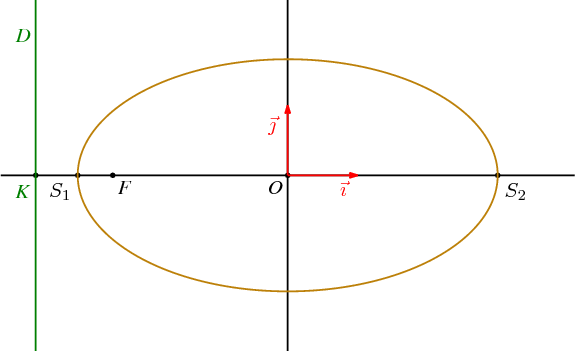Fichier 011.jps — Modifié le 11 Mars 2006 à 10 h 52Source
%% PhS
%%

50 setxunit
-5 5  setxrange
-2.5 2.5 setyrange

%%%%%%%%%%%%%%%%%%%%%%%%%%%%%%%%%%%%%%%%%%%%%%%
%% procédures
%%%%%%%%%%%%%%%%%%%%%%%%%%%%%%%%%%%%%%%%%%%%%%%

/bddroite {
8 dict begin
/kd exch def
/kg exch def
/By exch def
/Bx exch def
/B {Bx By} def
/Ay exch def
/Ax exch def
/A {Ax Ay} def
gsave
[A A B vecteur kg mulv addv A A B vecteur kd mulv addv] ligne
grestore
end
} def

%%%%%%%%%%%%%%%%%%%%%%%%%%%%%%%%%%%%%%%%%%%%%%%
%% definition d'une couleur
%%%%%%%%%%%%%%%%%%%%%%%%%%%%%%%%%%%%%%%%%%%%%%%

/coul1 {120 255 div 70 255 div 9 255 div setrgbcolor} def

%%%%%%%%%%%%%%%%%%%%%%%%%%%%%%%%%%%%%%%%%%%%%%%
%% definition des points
%%%%%%%%%%%%%%%%%%%%%%%%%%%%%%%%%%%%%%%%%%%%%%%

/a {3} def
/c {2.5} def
/b {a a mul c c mul sub sqrt} def
/e {c a div} def
/Kx {a e div} def
/K {Kx neg 0} def
/K+ {K 0 6 addv} def
/K- {K 0 -6 addv} def
/Kp {Kx 0} def
/Kp+ {Kp 0 6 addv} def
/Kp- {Kp 0 -6 addv} def
/O {0 0} def
/F {c neg 0} def
/Fp {c 0} def
/S1 {a neg 0} def
/S2 {a 0} def
/B {0 b} def

/xdet {settvar t cos a mul} def
/ydet {settvar t sin b mul} def

/Mx {60 xdet} def
/My {60 ydet} def
/M {Mx My} def
/H {M Kp Kp+ orthoproj} def

/bissec {F M Fp bissectrice} def
/bixx {bissec O S2 interdroite}def
/Mbixx {M bixx vecteur} def
/T {bissec M perp  Kp Kp+ interdroite} def
/MT {M T vecteur} def

/theta1 {c neg a div arccos} def
/Fcons1 {theta1 xdet theta1 ydet} def
/Fcons2 {theta1 neg xdet theta1 neg ydet} def

%%%%%%%%%%%%%%%%%%%%%%%%%%%%%%%%%%%%%%%%%%%%%%%
%% traces
%%%%%%%%%%%%%%%%%%%%%%%%%%%%%%%%%%%%%%%%%%%%%%%
/coul1 {188 255 div 128 255 div 9 255 div setrgbcolor} def

1.2 setlinewidth
[O F S1 S2 K] points

coul1
0 360 settrange
{xdet} {ydet} courbeparam

vert
[K+ K-] ligne

rouge
[0 0 1 0] (->) ligne
[0 0 0 1] (->) ligne

%%%%%%%%%%%%%%%%%%%%%%%%%%%%%%%%%%%%%%%%%%%%%%%
%% texte
%%%%%%%%%%%%%%%%%%%%%%%%%%%%%%%%%%%%%%%%%%%%%%%

setTimesItalic
noir
(O) O [1.5 dup] dltext
(F) F [1.5 dup] drtext

<latex>
$S_1$
</latex>
S1 [1.5 dup] dltexlabel

<latex>
$S_2$
</latex>
S2 [1.5 dup] drtexlabel

vert
(K) K (0 -3) [1.5 dup] dltext
(D) K 0 2 addv [1.5 dup] cltext

rouge
<latex>
$\vec{\imath}$
</latex>
0.8 -0.2 [1.5 dup] cctexlabel

<latex>
$\vec{\jmath}$
</latex>
-0.2 0.7 [1.5 dup] cctexlabel